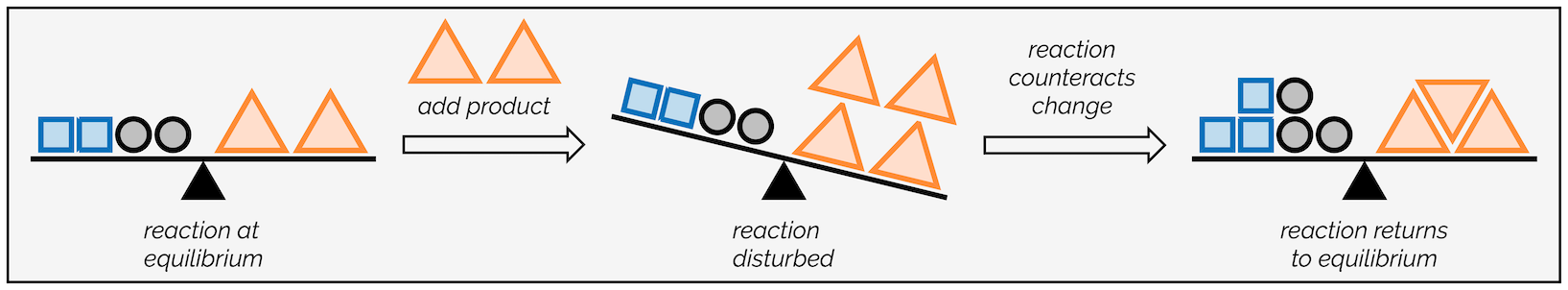Request Tutoring Info

## General Chemistry II Concept 1: Le Chatelier's Principle (Concentration Changes)

What is Le Chatelier's Principle?

Le Chatelier's Principle is, by definition, a law that explains that the position of equilibrium shifts to counteract changes done to a system to reestablish equilibrium. Simply put, it is a principle that explains the effects of change on a system.What kind of changes can be done to a system at equilibrium?

There are a few changes that can be made to either the concentration, temperature, or pressure.

If a chemical reaction at equilibrium experiences a change in concentration, temperature, or pressure of products or reactants, the equilibrium shifts in the opposite direction to offset any changes. Any action that changes the concentration, temperature, or pressure in a system at equilibrium will elicit a response that somewhat offsets the change while a new equilibrium condition is established. It is important to consider these changes in order for a reaction to reestablish equilibrium.

Note: It is important to understand that Le Chatelier's principle is only useful to identify what is happening when the conditions of a dynamic equilibrium system are changed. It does NOT give any reasons for any molecular changes or reaction mechanisms.

In this blog post, I will be discussing how a chemical reaction at equilibrium responds to changes in the concentration of reactants to reestablish a new equilibrium condition.

Concentration Changes:

According to Le Chatelier's Principle, if there is an increase in the concentration of one reacting species, then the species consumed is favored. So, if there is an increase in products, then the equilibrium shifts to favor the reactants. Let us consider a generic equilibrium equation established between 4 substances A, B, C, and D.(Equation 1)

Scenario 1: Increasing Concentration

Let us consider scenario 1. If we alter the conditions, so the concentration of A is increased then what happens to the equilibrium?

According to Le Chatelier's Principle, the equilibrium position will move to counteract the increase in the concentration of A. So, the equilibrium position will move to the right towards the products (C and D). The equilibrium position will move to the right so that the concentration of A will decrease by reacting with B in order to form more products (C and D). The equilibrium will shift in the direction indicated by the green arrow.(Equation 1 with an increase in the concentration of A)

Equilibrium shifts to the right towards the products to counteract the increase in the concentration of A.

Scenario 2: Decreasing Concentration

Now, let us observe the opposite scenario of the first condition discussed above. If we decrease the concentration of A, then according to Le Chatelier's Principle, the position of equilibrium will shift to the left in order for the concentration of A to increase. More products of C and D will react in order to replace the A that has been removed. The equilibrium will shift in the direction indicated by the green arrow.(Equation 1 with a decrease in the concentration of A)

Summary:

Change in concentration is just one possible change to a chemical equilibrium system. Depending on whether the products or the reactants are increased or decreased, then the equilibrium will shift in order to counteract any changes done to the equilibrium system.

1. Increase in Reactant Concentration = Equilibrium shifts to the Right / Towards the Product side of the reaction

2. Decrease in Reactant Concentration = Equilibrium shifts to the Left / Towards the Reactant side of the reaction

3. Increase in Product Concentration = Equilibrium shifts to the Left / Towards the Reactant side of the reaction

4. Decrease in the Product Concentration = Equilibrium shifts to the Right / Towards the Product side of the reaction

Le Chatelier's principles also account for changes in temperature and pressure. In the next blog series, I will discuss how temperature impacts the chemical equilibrium of a system. I will then discuss in detail how pressure impacts equilibrium. Finally, I will explain if or if not catalysts impact equilibrium, and finally I will define the reaction quotient, Q. With the introduction of the reaction quotient, Q, I will show mathematically how each condition (change in concentration, pressure, and temperature) impacts the reaction quotient.

Eunice K
Well-Rounded Experienced Tutor
Oberlin College